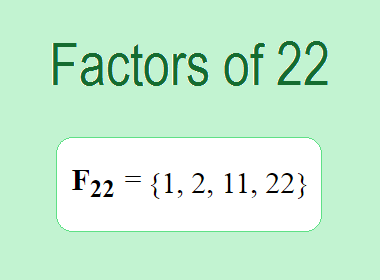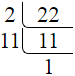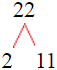# Factors of 22The factors of 22 are 1, 2, 11, and 22 i.e. F22 = {1, 2, 11, 22}. The factors of 22 are all the numbers that can divide 22 without leaving a remainder.

We can check if these numbers are factors of 22 by dividing 22 by each of them. If the result is a whole number, then the number is a factor of 22. Let's do this for each of the numbers listed above:

·        1 is a factor of 22 because 22 divided by 1 is 22.

·        2 is a factor of 22 because 22 divided by 2 is 11.

·        11 is a factor of 22 because 22 divided by 7 is 2.

·        22 is a factor of 22 because 22 divided by 22 is 1.

## How to Find Factors of 22?

1 and the number itself are the factors of every number. So, 1 and 22 are two factors of 22. To find the other factors of 22, we can start by dividing 22 by the numbers between 1 and 22. If we divide 22 by 2, we get a remainder of 0. Therefore, 2 is a factor of 22. If we divide 22 by 3, we get a remainder of 1. Therefore, 3 is not a factor of 22.

Next, we can check if 4 is a factor of 22. If we divide 22 by 4, we get a remainder of 2. Therefore, 4 is not a factor of 22. We can continue this process for all the possible factors of 22.

Through this process, we can find that the factors of 22 are 1, 2, 11, and 22. These are the only numbers that can divide 22 without leaving a remainder.

********************

********************

## Properties of the Factors of 22

The factors of 22 have some interesting properties. One of the properties is that the sum of the factors of 22 is equal to 36. We can see this by adding all the factors of 22 together:

1 + 2 + 11 + 22 = 36

Another property of the factors of 22 is that they are all prime numbers except 1 and 22.

## Applications of the Factors of 22

The factors of 22 have several applications in mathematics. One of the applications is in finding the highest common factor (HCF) of two or more numbers. The HCF is the largest factor that two or more numbers have in common. For example, to find the HCF of 22 and 33, we need to find the factors of both numbers and identify the largest factor they have in common. The factors of 22 are 1, 2, 11, and 22. The factors of 33 are 1, 3, 11, and 33. The largest factor that they have in common is 11. Therefore, the HCF of 22 and 33 is 11.

Another application of the factors of 22 is in prime factorization. Prime factorization is the process of expressing a number as the product of its prime factors. The prime factors of 22 are 2 and 11, since these are the only prime numbers that can divide 22 without leaving a remainder. Therefore, we can express 22 as:

22 = 2 × 11

We can do prime factorization by division and factor tree method also. Here is the prime factorization of 22 by division method,22 = 2 × 11

Here is the prime factorization of 22 by the factor tree method,22 = 2 × 11## Conclusion

The factors of 22 are the numbers that can divide 22 without leaving a remainder. The factors of 22 are 1, 2, 11, and 22. The factors of 22 have some interesting properties, such as having a sum of 36. The factors of 22 have several applications in mathematics, such as finding the highest common factor and prime factorization.